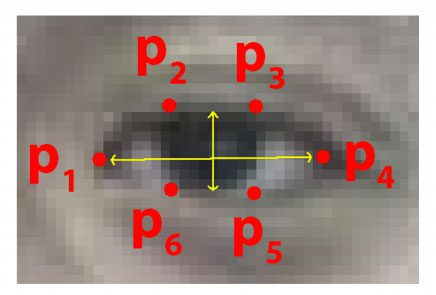# 基于Python OpenCV和 dlib实现眨眼检测

##### 目录

• 眼睛定位。
• 阈值以找到眼白。
• 确定眼睛的“白色”区域是否在一段时间内消失（表示眨眼）。
• 眼睛纵横比是一个更优雅的解决方案，它涉及基于眼睛面部标志之间距离比的非常简单的计算。## 了解“眼睛纵横比”（EAR）## 使用面部标志和 OpenCV 检测眨眼

 1234567891011 `# import the necessary packages``from` `scipy.spatial ``import` `distance as dist``from` `imutils.video ``import` `FileVideoStream``from` `imutils.video ``import` `VideoStream``from` `imutils ``import` `face_utils``import` `numpy as np``import` `argparse``import` `imutils``import` `time``import` `dlib``import` `cv2`

 1 `pip install ``-``-``upgrade imutils <``/``span><``/``font>`

接下来，我们将定义我们的 eye_aspect_ratio 函数：

 123456789101112 `def` `eye_aspect_ratio(eye):``    ``# compute the euclidean distances between the two sets of``    ``# vertical eye landmarks (x, y)-coordinates``    ``A ``=` `dist.euclidean(eye[``1``], eye[``5``])``    ``B ``=` `dist.euclidean(eye[``2``], eye[``4``])``    ``# compute the euclidean distance between the horizontal``    ``# eye landmark (x, y)-coordinates``    ``C ``=` `dist.euclidean(eye[``0``], eye[``3``])``    ``# compute the eye aspect ratio``    ``ear ``=` `(A ``+` `B) ``/` `(``2.0` `*` `C)``    ``# return the eye aspect ratio``    ``return` `ear`

 1234567 `# construct the argument parse and parse the arguments``ap ``=` `argparse.ArgumentParser()``ap.add_argument(``"-p"``, ``"--shape-predictor"``, required``=``True``,``    ``help``=``"path to facial landmark predictor"``)``ap.add_argument(``"-v"``, ``"--video"``, ``type``=``str``, default``=``"",``    ``help``=``"path to input video file"``)``args ``=` `vars``(ap.parse_args())`

1. –shape-predictor ：这是 dlib 的预训练面部标志检测器的路径。 您可以使用本博文底部的“下载”部分将检测器以及源代码 + 示例视频下载到本教程中。
2. –video ：此可选开关控制驻留在磁盘上的输入视频文件的路径。 如果您想使用实时视频流，只需在执行脚本时省略此开关即可。

 12345678 `# 定义两个常量，一个为眼睛纵横比来表示``# 闪烁然后第二个常量为连续的次数``# 帧眼睛必须低于阈值``EYE_AR_THRESH ``=` `0.3``EYE_AR_CONSEC_FRAMES ``=` `3``# 初始化帧计数器和闪烁总数``COUNTER ``=` `0``TOTAL ``=` `0`

 12345 `# 初始化dlib的人脸检测器（基于HOG）然后创建``# 面部标志预测器``print``(``"[INFO] loading facial landmark predictor..."``)``detector ``=` `dlib.get_frontal_face_detector()``predictor ``=` `dlib.shape_predictor(args[``"shape_predictor"``])`

dlib 生成的面部标志遵循可索引的列表，如下：1234 `# 获取左侧和面部标志的索引``# 右眼，分别 ``(lStart, lEnd) ``=` `face_utils.FACIAL_LANDMARKS_IDXS[``"left_eye"``]``(rStart, rEnd) ``=` `face_utils.FACIAL_LANDMARKS_IDXS[``"right_eye"``]`

 1234567891011 `# start the video stream thread``print``(``"[INFO] starting video stream thread..."``)``vs ``=` `FileVideoStream(args[``"video"``]).start()``fileStream ``=` `True``# vs = VideoStream(src=0).start()``# vs = VideoStream(usePiCamera=True).start()``# fileStream = False``time.sleep(``1.0``)``fps ``=` `30`    `#保存视频的FPS，可以适当调整``size``=``(``450``,``800``)``videoWriter ``=` `cv2.VideoWriter(``'3.mp4'``,``-``1``,fps,size)``#最后一个是保存图片的尺寸`

 12345678910111213 `# loop over frames from the video stream``while` `True``:``    ``# 如果这是一个文件视频流，那么我们需要检查是否``    ``# 缓冲区中还有更多帧要处理``    ``if` `fileStream ``and` `not` `vs.more():``        ``break``    ``frame ``=` `vs.read()``    ``if` `frame ``is` `None``:``        ``break``    ``frame ``=` `imutils.resize(frame, width``=``450``)``    ``gray ``=` `cv2.cvtColor(frame, cv2.COLOR_BGR2GRAY)``    ``# 在灰度帧中检测人脸``    ``rects ``=` `detector(gray, ``0``)`

 1234567891011121314 `# loop over the face detections``for` `rect ``in` `rects:``    ``# 确定面部区域的面部标志，然后``    ``# 将面部标志 (x, y) 坐标转换为 NumPy数组``    ``shape ``=` `predictor(gray, rect)``    ``shape ``=` `face_utils.shape_to_np(shape)``    ``# 提取左右眼坐标，然后使用``    ``# 坐标来计算双眼的眼睛纵横比``    ``leftEye ``=` `shape[lStart:lEnd]``    ``rightEye ``=` `shape[rStart:rEnd]``    ``leftEAR ``=` `eye_aspect_ratio(leftEye)``    ``rightEAR ``=` `eye_aspect_ratio(rightEye)``    ``# 平均两只眼睛的眼睛纵横比``    ``ear ``=` `(leftEAR ``+` `rightEAR) ``/` `2.0`

 123456 `# 计算左眼和右眼的凸包，然后``# 可视化每只眼睛``leftEyeHull ``=` `cv2.convexHull(leftEye)``rightEyeHull ``=` `cv2.convexHull(rightEye)``cv2.drawContours(frame, [leftEyeHull], ``-``1``, (``0``, ``255``, ``0``), ``1``)``cv2.drawContours(frame, [rightEyeHull], ``-``1``, (``0``, ``255``, ``0``), ``1``)`

 12345678910111213 `# 检查眼睛的纵横比是否低于眨眼``# 阈值，如果是，则增加闪烁帧计数器``if` `ear < EYE_AR_THRESH:``    ``COUNTER ``+``=` `1``# 否则，眼睛纵横比不低于眨眼``＃ 临界点``else``:``    ``# 如果眼睛闭上足够多的次数``    ``# 然后增加闪烁的总数``    ``if` `COUNTER >``=` `EYE_AR_CONSEC_FRAMES:``        ``TOTAL ``+``=` `1``    ``# 重置眼框计数器``    ``COUNTER ``=` `0`

 12345678910111213141516171819 `        ``# 绘制帧上闪烁的总数以及``        ``# 计算出的帧的眼睛纵横比``        ``cv2.putText(frame, ``"Blinks: {}"``.``format``(TOTAL), (``10``, ``30``),``            ``cv2.FONT_HERSHEY_SIMPLEX, ``0.7``, (``0``, ``0``, ``255``), ``2``)``        ``cv2.putText(frame, ``"EAR: {:.2f}"``.``format``(ear), (``300``, ``30``),``            ``cv2.FONT_HERSHEY_SIMPLEX, ``0.7``, (``0``, ``0``, ``255``), ``2``)`` ` `    ``# show the frame``    ``cv2.imshow(``"Frame"``, frame)``    ``videoWriter.write(frame)``    ``key ``=` `cv2.waitKey(``1``) & ``0xFF`` ` `    ``# if the `q` key was pressed, break from the loop``    ``if` `key ``=``=` `ord``(``"q"``):``        ``break``videoWriter.release()``# do a bit of cleanup``cv2.destroyAllWindows()``vs.stop()`

## 眨眼检测结果

 1 `python detect_blinks.py ``-``-``shape``-``predictor shape_predictor_68_face_landmarks.dat ``-``-``video ``11.mp4`12345 `#vs = FileVideoStream(args["video"]).start()``#fileStream = True``vs ``=` `VideoStream(src``=``0``).start()``# vs = VideoStream(usePiCamera=True).start()``fileStream ``=` `False`

 1 `python detect_blinks.py ``-``-``shape``-``predictor shape_predictor_68_face_landmarks.dat`